# Acceleration Circular Motion Centripetal Acceleration Tangential Acceleration Centripetal

• Slides: 26Acceleration & Circular Motion Centripetal Acceleration Tangential AccelerationCentripetal acceleration ac tangential acceleration is how fast the speed changes Centripetal acceleration is how fast the velocity changes because of its direction change.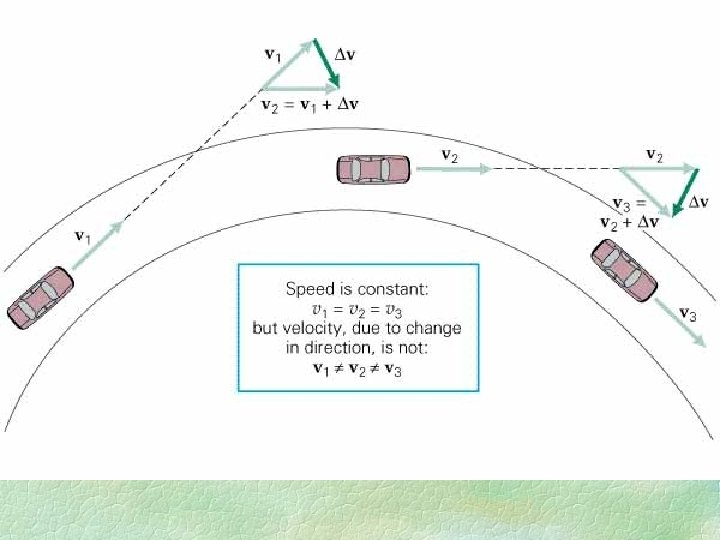Centripetal acceleration ac vf =vo +∆v vf = vo = v vf voCentripetal acceleration ac vf =vo +∆v vf = vo = v vo vfCentripetal acceleration ac vf =vo +∆v vf vf = vo = v ∆v r q r s vf q vo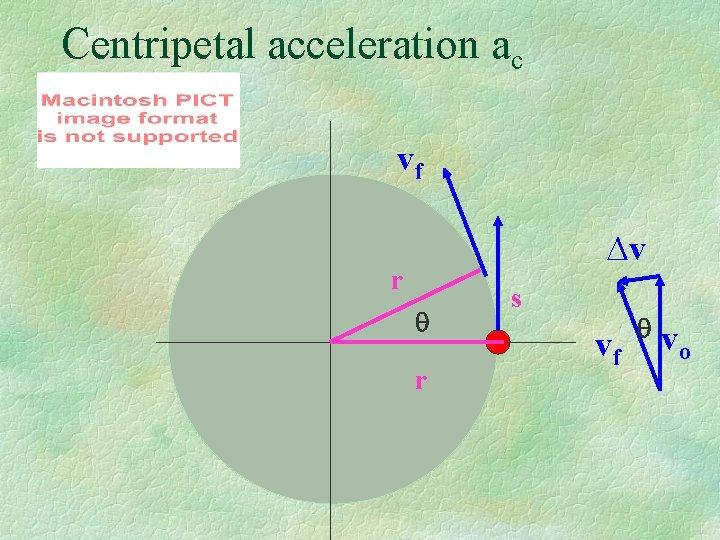Centripetal acceleration ac vf ∆v r q r s vf q vo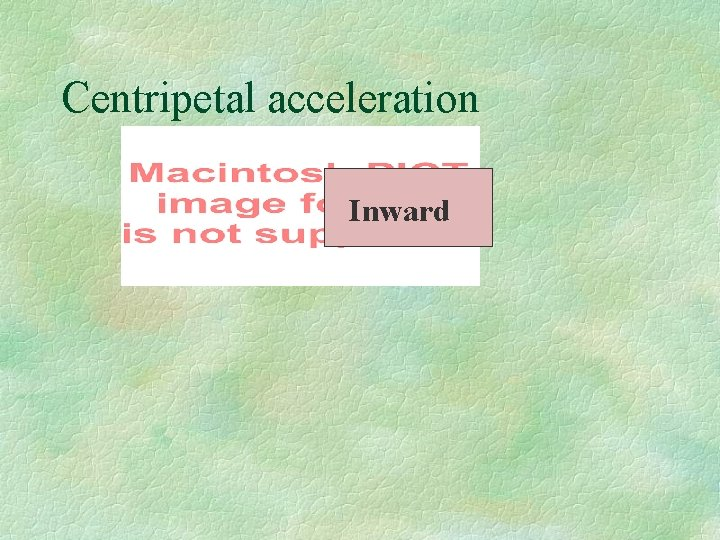Centripetal acceleration Inward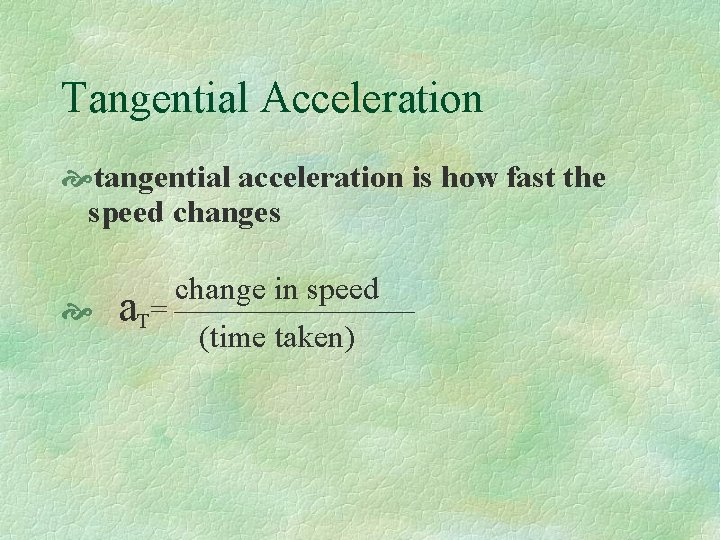Tangential Acceleration tangential acceleration is how fast the speed changes change in speed a T= (time taken)Fig. 4. 18, p. 94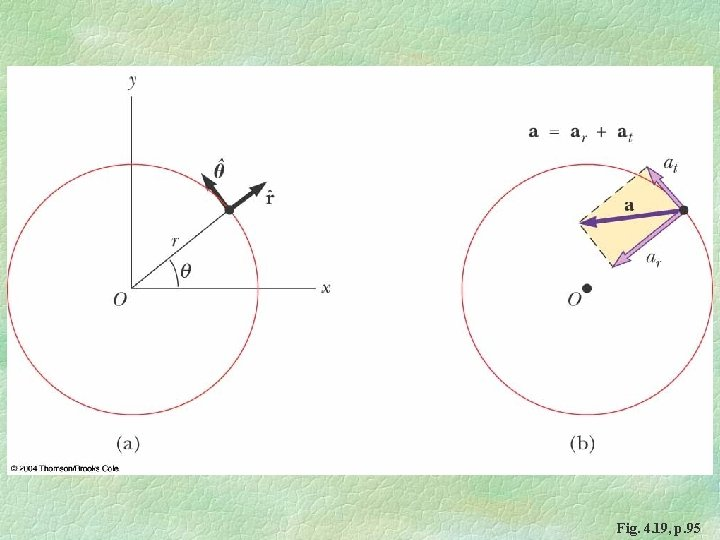Fig. 4. 19, p. 95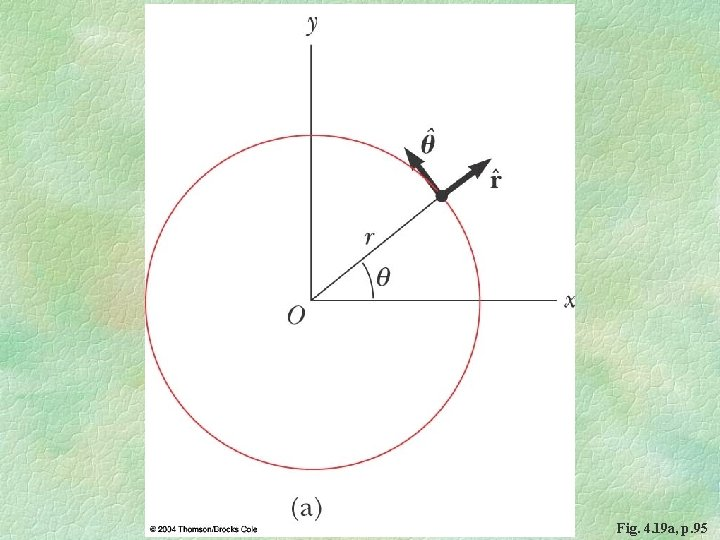Fig. 4. 19 a, p. 95Fig. 4. 19 b, p. 95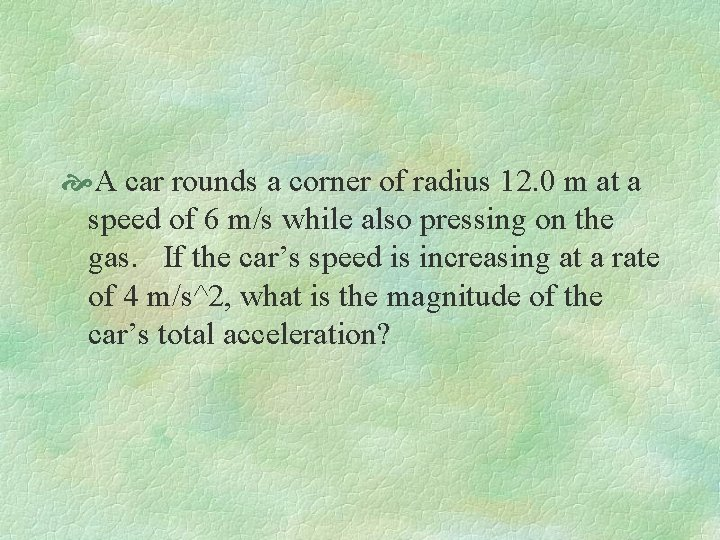A car rounds a corner of radius 12. 0 m at a speed of 6 m/s while also pressing on the gas. If the car’s speed is increasing at a rate of 4 m/s^2, what is the magnitude of the car’s total acceleration?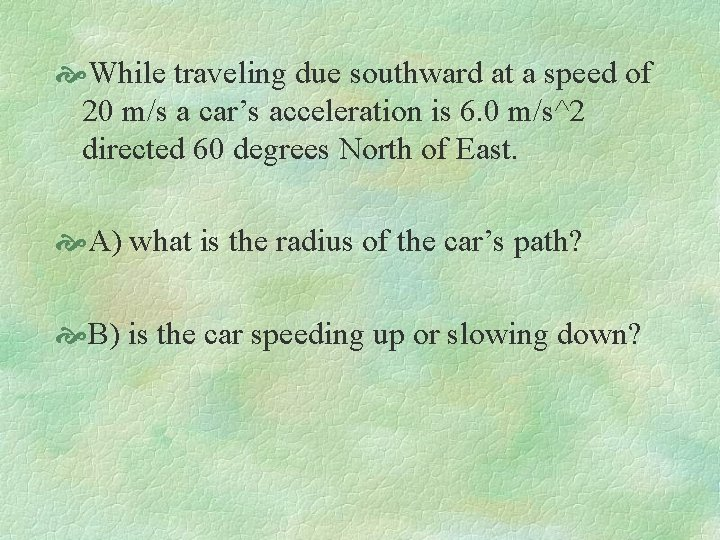While traveling due southward at a speed of 20 m/s a car’s acceleration is 6. 0 m/s^2 directed 60 degrees North of East. A) what is the radius of the car’s path? B) is the car speeding up or slowing down?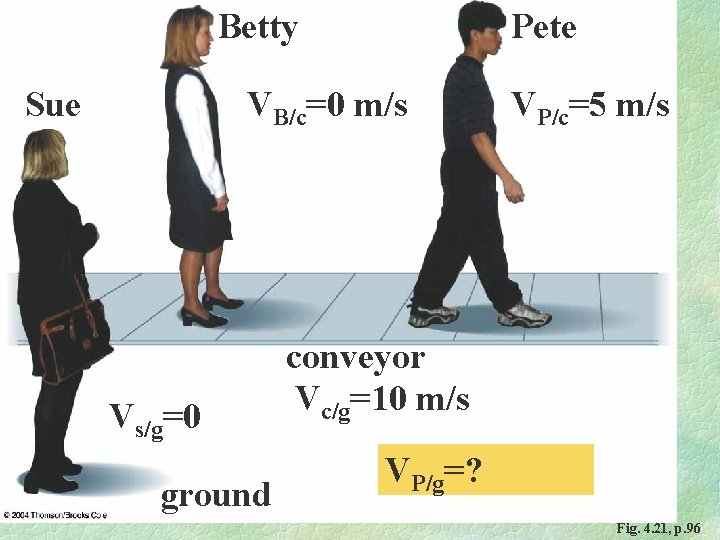Betty Sue Pete VB/c=0 m/s Vs/g=0 ground VP/c=5 m/s conveyor Vc/g=10 m/s VP/g=? Fig. 4. 21, p. 96Betty Sue VB/c=0 m/s Vs/g=0 ground Pete VP/c=5 m/s conveyor Vc/g=10 m/s VP/g= VP/c+ Vc/g=15 m/s Fig. 4. 21, p. 96Betty Sue Pete VB/c=10 m/s Vs/g=0 ground VP/c=-5 m/s conveyor Vc/g=10 m/s VP/B=? Fig. 4. 21, p. 96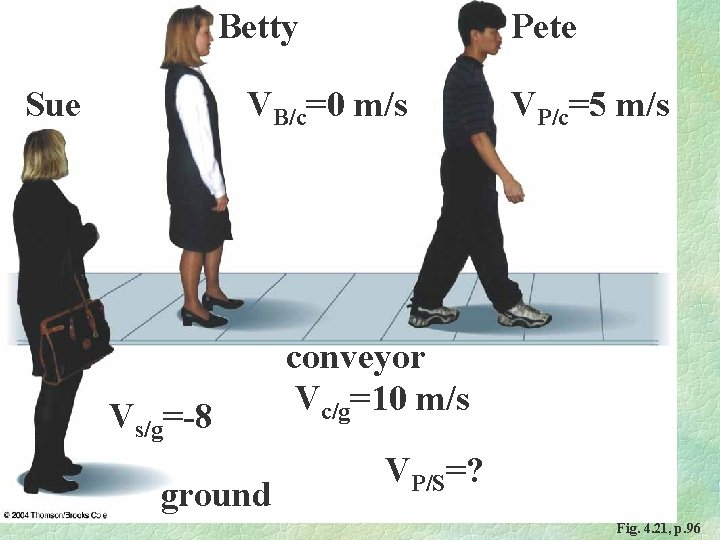Betty Sue Pete VB/c=0 m/s Vs/g=-8 ground VP/c=5 m/s conveyor Vc/g=10 m/s VP/S=? Fig. 4. 21, p. 96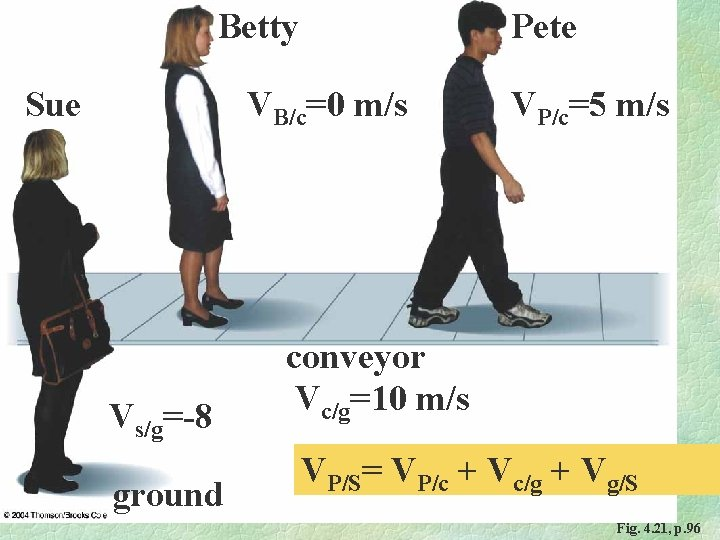Betty Sue Pete VB/c=0 m/s Vs/g=-8 ground VP/c=5 m/s conveyor Vc/g=10 m/s VP/S= VP/c + Vc/g + Vg/S Fig. 4. 21, p. 96Fig. 4. 22, p. 97Fig. 4. 22 a, p. 97Fig. 4. 22 b, p. 97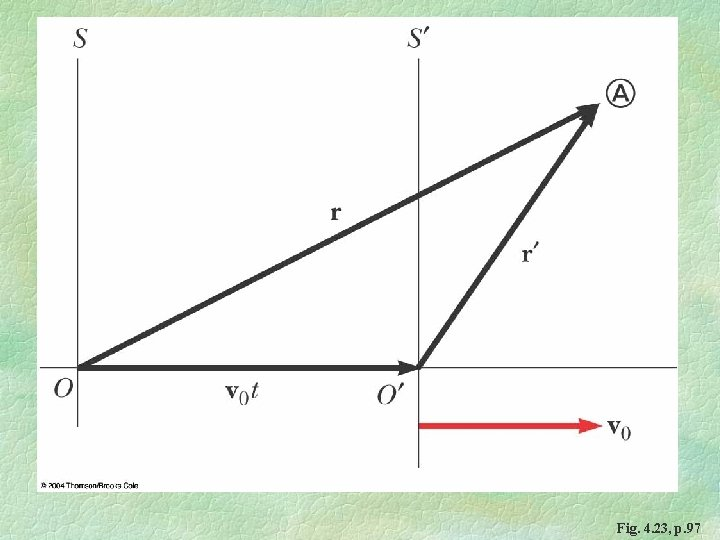Fig. 4. 23, p. 97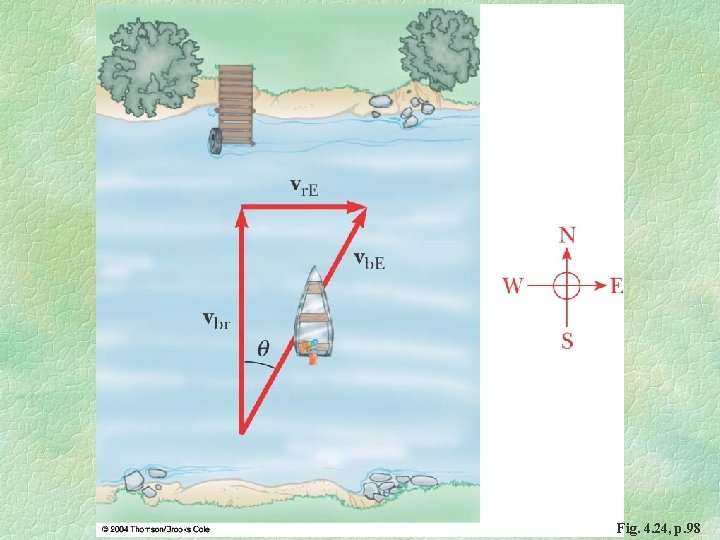Fig. 4. 24, p. 98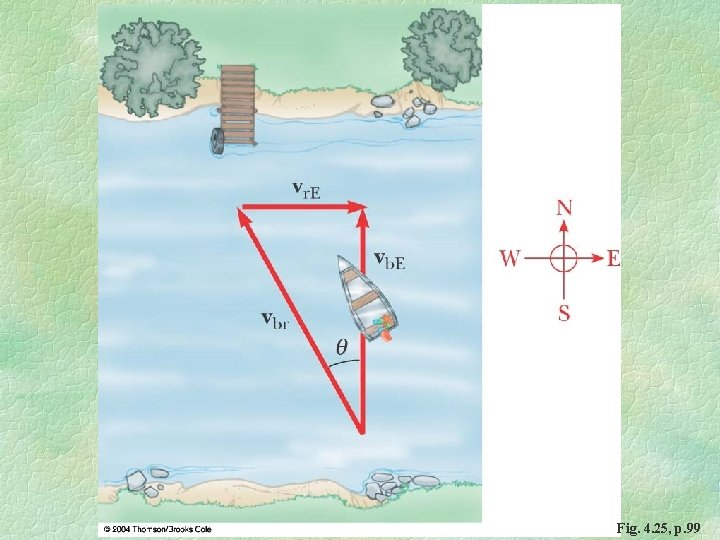Fig. 4. 25, p. 99